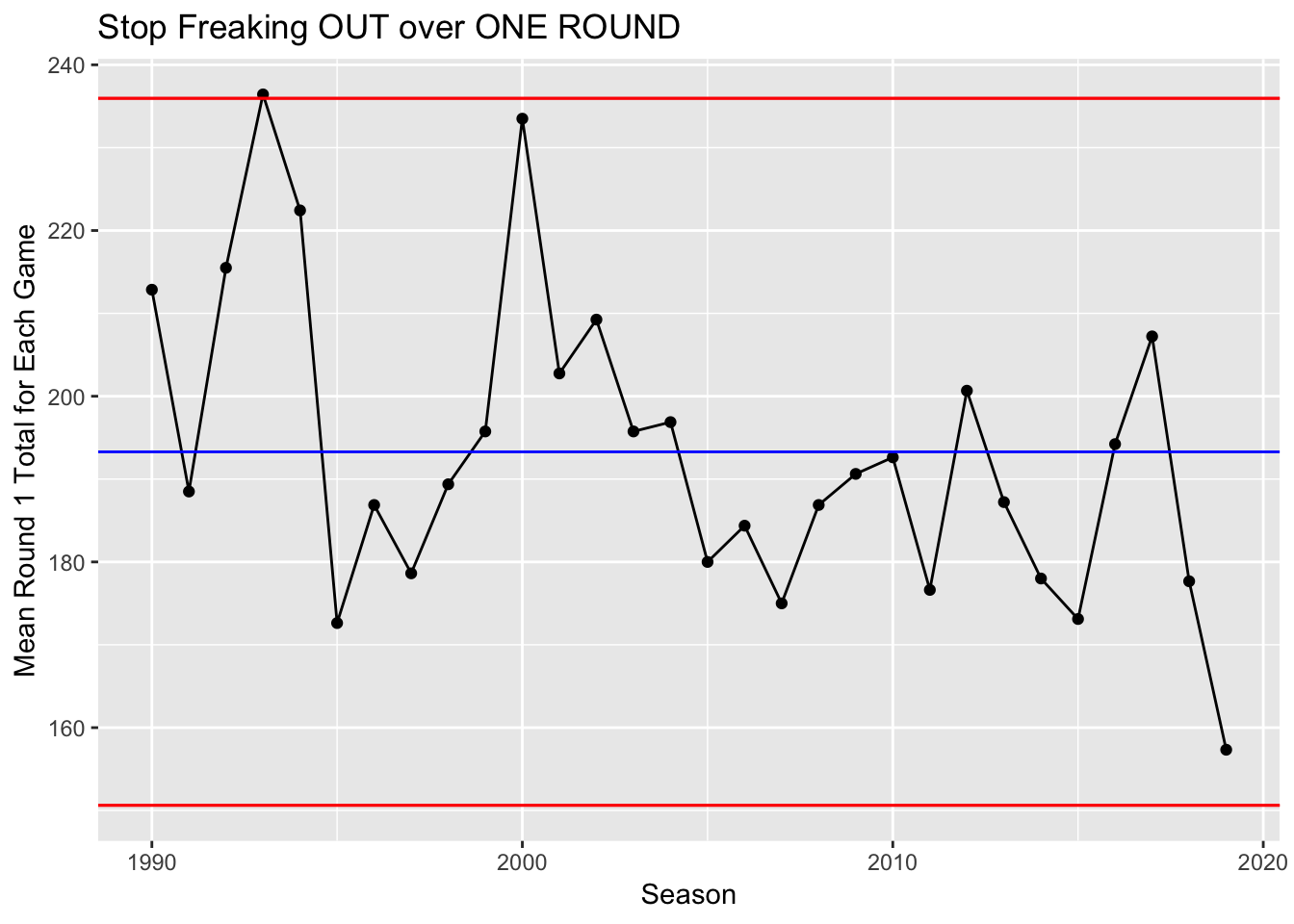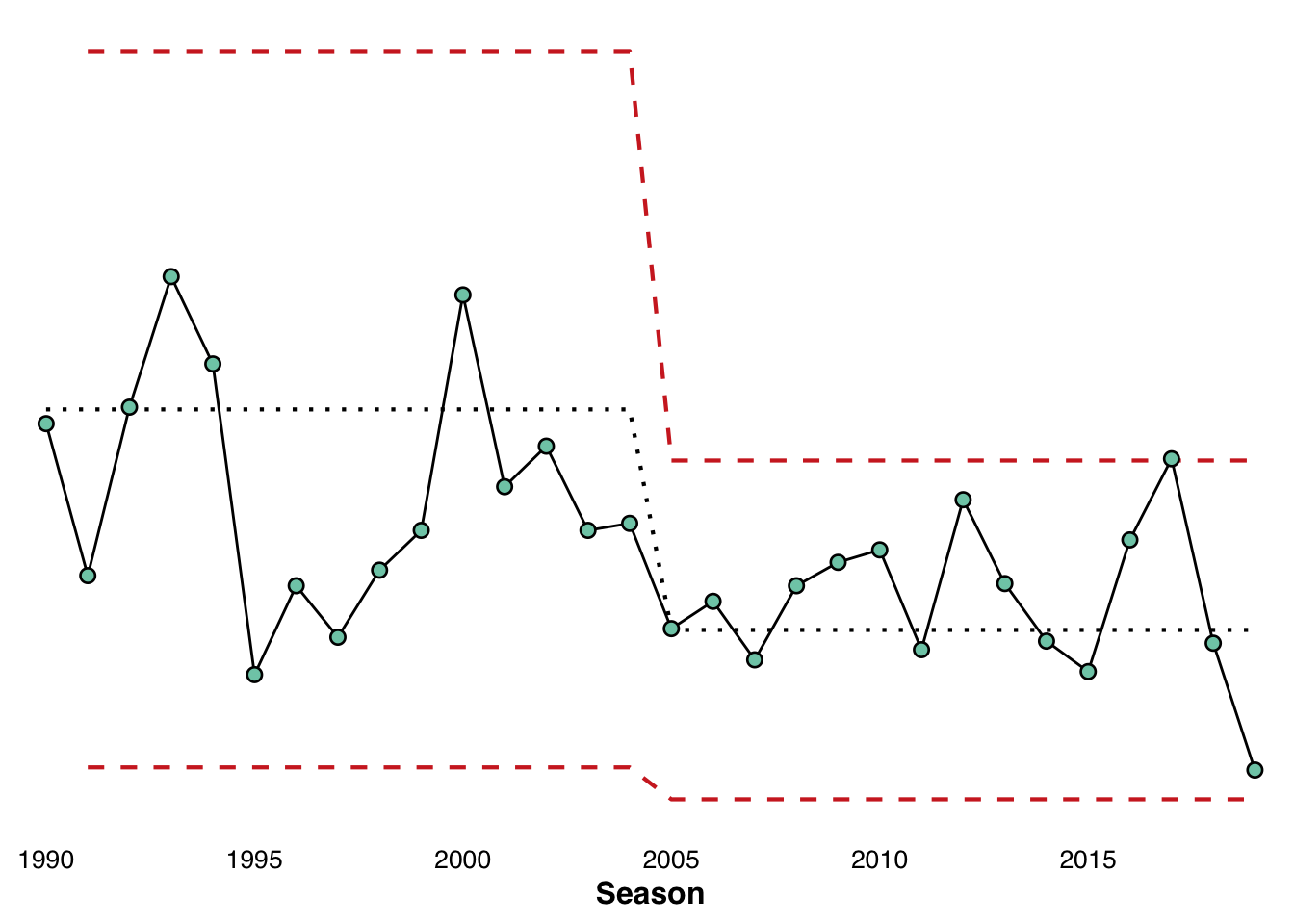I got an email from Alex Zanidean, who runs the xmrr package

“You might enjoy my package xmrr for similar charts – but mine recalculate the bounds automatically” and if we go to the vingette, “XMRs combine X-Bar control charts and Moving Range control charts. These functions also will recalculate the reference lines when significant change has occurred” This seems like a pretty handy thing. So lets do it.

First lets do our graphic from our previous post using ggQC

library(fitzRoy)
library(tidyverse)
## ── Attaching packages ───── tidyverse 1.2.1 ──
## ✔ ggplot2 3.1.1       ✔ purrr   0.3.2
## ✔ tibble  2.1.1       ✔ dplyr   0.8.0.1
## ✔ tidyr   0.8.3       ✔ stringr 1.4.0
## ✔ readr   1.3.1       ✔ forcats 0.4.0
## ── Conflicts ──────── tidyverse_conflicts() ──
## ✖ dplyr::lag()    masks stats::lag()
library(ggQC)
library(xmrr)
fitzRoy::match_results%>%
mutate(total=Home.Points+Away.Points)%>%
group_by(Season,Round)%>%
summarise(meantotal=mean(total))%>%
filter(Season>1989 &  Round=="R1")%>%
ggplot(aes(x=Season,y=meantotal))+geom_point()+
geom_line()+stat_QC(method="XmR")+
ylab("Mean Round 1 Total for Each Game") +ggtitle("Stop Freaking OUT over ONE ROUND")df<-fitzRoy::match_results%>%
mutate(total=Home.Points+Away.Points)%>%
group_by(Season,Round)%>%
summarise(meantotal=mean(total))%>%
filter(Season>1989 &  Round=="R1")

So when using a package for the first time, one of the best things about the R community is how the examples are usually fully reproducible and this helps.

From the github

Year <- seq(2001, 2009, 1)
Measure <-  runif(length(Year))

df <- data.frame(Year, Measure)
head(df)
##   Year   Measure
## 1 2001 0.5032418
## 2 2002 0.7435385
## 3 2003 0.1127812
## 4 2004 0.3818857
## 5 2005 0.6752043
## 6 2006 0.4476480
xmr(df, "Measure", recalc = T)
##   Year   Measure Order Central Line Moving Range Average Moving Range
## 1 2001 0.5032418     1        0.483           NA                   NA
## 2 2002 0.7435385     2        0.483        0.240                0.358
## 3 2003 0.1127812     3        0.483        0.631                0.358
## 4 2004 0.3818857     4        0.483        0.269                0.358
## 5 2005 0.6752043     5        0.483        0.293                0.358
## 6 2006 0.4476480     6        0.483        0.228                0.358
## 7 2007 0.3416156     7        0.483        0.106                0.358
## 8 2008 0.3271194     8        0.483        0.014                0.358
## 9 2009 0.5749751     9        0.483        0.248                0.358
##   Lower Natural Process Limit Upper Natural Process Limit
## 1                          NA                          NA
## 2                           0                       1.437
## 3                           0                       1.437
## 4                           0                       1.437
## 5                           0                       1.437
## 6                           0                       1.437
## 7                           0                       1.437
## 8                           0                       1.437
## 9                           0                       1.437

Lets create a similar dataframe as df, but using data from fitzRoy

df<-fitzRoy::match_results%>%
mutate(total=Home.Points+Away.Points)%>%
group_by(Season,Round)%>%
summarise(meantotal=mean(total))%>%
filter(Season>1989 &  Round=="R1")%>%
select(Season, meantotal)
df<-data.frame(df)
xmr_data <-xmr(df, "meantotal", recalc = T)

xmr_chart(df = xmr_data,
time = "Season",
measure = "meantotal",
line_width = 0.75, text_size = 12, point_size = 2.5) +
scale_x_discrete(breaks = seq(1990, 2020, 5))Does this tell a different story or a very similar one to earlier?